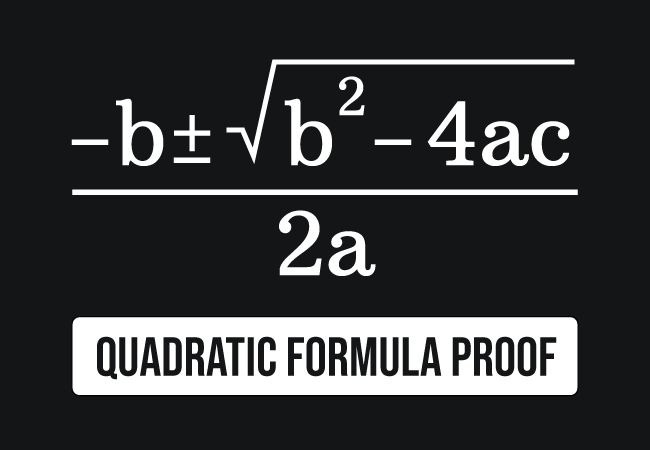$ax^2+bx+c = 0$ is a standard algebraic form of a quadratic equation in mathematics and the solution of this general form equation is expressed by the following quadratic formula.

$x \,=\, \dfrac{-b \pm \sqrt{b^2-4ac}}{2a}$

Now, let’s learn how to derive the quadratic formula fundamentally in mathematics. It is actually proved by completing the square method.

### Shift the constant to other side of equation

The term $c$ represents the constant. Now, move the constant $c$ to the right side of the equation.

$\implies$ $ax^2+bx \,=\, -c$

### Use completing the square technique

For converting the left side expression of the equation into square form of a binomial completely, eliminate the coefficient of $x^2$ from the first term. So, divide both sides of the equation by $a$.

$\implies$ $\dfrac{ax^2+bx}{a}$ $\,=\,$ $-\dfrac{c}{a}$

$\implies$ $\dfrac{ax^2}{a}+\dfrac{bx}{a}$ $\,=\,$ $-\dfrac{c}{a}$

$\implies$ $\bigg(\dfrac{a}{a}\bigg)x^2+\bigg(\dfrac{b}{a}\bigg)x$ $\,=\,$ $-\dfrac{c}{a}$

$\implies$ $\bigg(\dfrac{\cancel{a}}{\cancel{a}}\bigg)x^2+\bigg(\dfrac{b}{a}\bigg)x$ $\,=\,$ $-\dfrac{c}{a}$

$\implies$ $x^2+\bigg(\dfrac{b}{a}\bigg)x$ $\,=\,$ $-\dfrac{c}{a}$

Now, the left hand side expression of the equation should be transformed into complete square form. It can be obtained by adjusting the second term slightly for our convenience.

$\implies$ $x^2+1 \times \bigg(\dfrac{b}{a}\bigg)x$ $\,=\,$ $-\dfrac{c}{a}$

The multiplying factor $1$ can be written as the quotient of $2$ by $2$ because the division of $2$ by $2$ is $1$.

$\implies$ $x^2+\bigg(\dfrac{2}{2}\bigg) \times \bigg(\dfrac{b}{a}\bigg)x$ $\,=\,$ $-\dfrac{c}{a}$

$\implies$ $x^2$ $+$ $2 \times \dfrac{1}{2} \times \bigg(\dfrac{b}{a}\bigg)x$ $\,=\,$ $-\dfrac{c}{a}$

$\implies$ $x^2$ $+$ $2 \times \bigg(\dfrac{b}{2a}\bigg)x$ $\,=\,$ $-\dfrac{c}{a}$

$\implies$ $x^2$ $+$ $2 \times \bigg(\dfrac{b}{2a}\bigg) \times x$ $\,=\,$ $-\dfrac{c}{a}$

$\implies$ $x^2$ $+$ $2 \times x \times \bigg(\dfrac{b}{2a}\bigg)$ $\,=\,$ $-\dfrac{c}{a}$

$\implies$ $x^2$ $+$ $2x\bigg(\dfrac{b}{2a}\bigg)$ $\,=\,$ $-\dfrac{c}{a}$

There is a chance to transform the left hand side expression as a square of sum of two terms but the expression should contain a term $\bigg(\dfrac{b}{2a}\bigg)^2$. Thereore, add $\bigg(\dfrac{b}{2a}\bigg)^2$ to the expression and also subtract it from the same expression.

$\implies$ $x^2$ $+$ $2x\bigg(\dfrac{b}{2a}\bigg)$ $+$ $\bigg(\dfrac{b}{2a}\bigg)^2$ $-$ $\bigg(\dfrac{b}{2a}\bigg)^2$ $\,=\,$ $-\dfrac{c}{a}$

Now, move the negative constant quantity to the right hand side expression of the equation.

$\implies$ $x^2$ $+$ $2x\bigg(\dfrac{b}{2a}\bigg)$ $+$ $\bigg(\dfrac{b}{2a}\bigg)^2$ $\,=\,$ $\bigg(\dfrac{b}{2a}\bigg)^2$ $-$ $\dfrac{c}{a}$

The left hand side expression of the equation represents the expansion of the square of sum of two terms formula. So, it can be converted to a square of a binomial completely by this rule.

$\implies$ $\bigg(x+\dfrac{b}{2a}\bigg)^2$ $\,=\,$ $\bigg(\dfrac{b}{2a}\bigg)^2$ $-$ $\dfrac{c}{a}$

### Solve the equation by simplification

The simplification process can be started by simplifying the right hand side expression of the equation. The first term can be simplified by the power of a quotient rule.

$\implies$ $\bigg(x+\dfrac{b}{2a}\bigg)^2$ $\,=\,$ $\dfrac{b^2}{(2a)^2}$ $-$ $\dfrac{c}{a}$

$\implies$ $\bigg(x+\dfrac{b}{2a}\bigg)^2$ $\,=\,$ $\dfrac{b^2}{4a^2}$ $-$ $\dfrac{c}{a}$

Now, use the subtraction of the fractions to find the difference of them mathematically.

$\implies$ $\bigg(x+\dfrac{b}{2a}\bigg)^2$ $\,=\,$ $\dfrac{b^2-4ac}{4a^2}$

Take square root to both sides of the equation for eliminating the square of the left hand side expression in the above equation.

$\implies$ $x+\dfrac{b}{2a}$ $\,=\,$ $\pm \sqrt{\dfrac{b^2-4ac}{4a^2}}$

$\implies$ $x+\dfrac{b}{2a}$ $\,=\,$ $\pm \sqrt{\dfrac{b^2-4ac}{{(2a)}^2}}$

$\implies$ $x+\dfrac{b}{2a}$ $\,=\,$ $\pm \dfrac{\sqrt{b^2-4ac}}{2a}$

$\implies$ $x \,=\, -\dfrac{b}{2a} \pm \dfrac{\sqrt{b^2-4ac}}{2a}$

$\,\,\, \therefore \,\,\,\,\,\, x \,=\, \dfrac{-b \pm \sqrt{b^2-4ac}}{2a}$

In this way, the quadratic formula is derived mathematically by completing the square method in mathematics.

Latest Math Topics
Jun 26, 2023
Jun 23, 2023

###### Math Questions

The math problems with solutions to learn how to solve a problem.

Learn solutions

Practice now

###### Math Videos

The math videos tutorials with visual graphics to learn every concept.

Watch now

###### Subscribe us

Get the latest math updates from the Math Doubts by subscribing us.